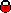## Forward neutron PT distributions

 Reference Preliminary ICHEP2010, July 2010 Figures (1)  (2)  (3) Abstract The production of leading neutrons, where the neutron carries a large fraction x_L of the incoming proton's longitudinal momentum, is studied in deep-inelastic positron-proton scattering at HERA. The data are taken with the H1 detector in the years 2006 and 2007 and correspond to an integrated luminosity of 122 pb-1. The semi-inclusive double differential cross sections are measured as function of a neutron longitudinal momentum fraction x_L and neutron transverse momentum sqaured p_T^2 in the phase space defined by the photon virtuality 6 < Q2 < 100 GeV2, the inelasticity 0.05 < y < 0.6, the longitudinal momentum fraction 0.32 < xL < 1.00 and transverse momentum p_T < xL*0.69 GeV of the neutron. The slopes of p_T^2 distributions are extracted in bins of x_L and are compared to different pion flux parameterisations. Links back to overview listingH1 internal information